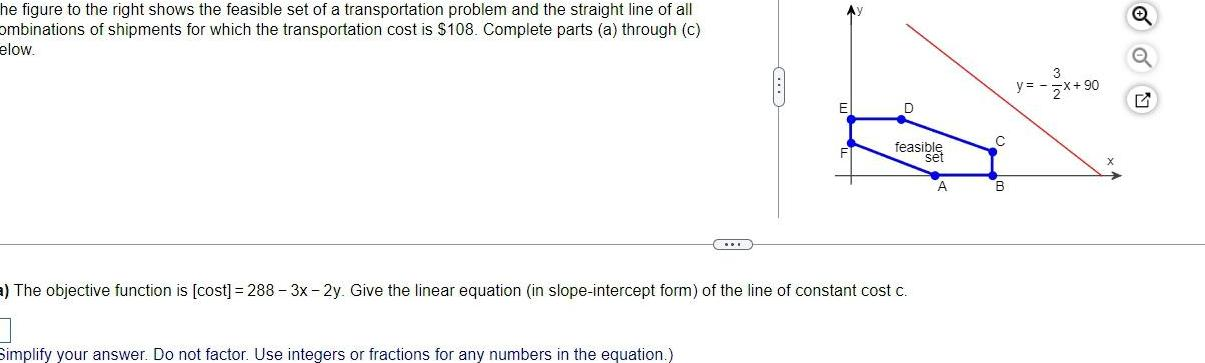Question:

# he figure to the right shows the feasible set of a

Last updated: 9/16/2023he figure to the right shows the feasible set of a transportation problem and the straight line of all ombinations of shipments for which the transportation cost is 108 Complete parts a through c elow D feasible set a The objective function is cost 288 3x 2y Give the linear equation in slope intercept form of the line of constant cost c 3 Simplify your answer Do not factor Use integers or fractions for any numbers in the equation A C B y x 0 Q